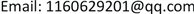﻿ 一类混合束方法子问题的求解研究 Study on the Solution of a Hybrid Bundle Method Subproblem

Operations Research and Fuzziology
Vol. 09  No. 02 ( 2019 ), Article ID: 30501 , 5 pages
10.12677/ORF.2019.92019

Study on the Solution of a Hybrid Bundle Method Subproblem

Hanyang Li

School of Mathematics, Liaoning Normal University, Dalian LiaoningReceived: May 8th, 2019; accepted: May 22nd, 2019; published: May 29th, 2019ABSTRACT

The solutions of non-smooth optimization problem have been the focus of the optimization theory for a long time. This paper proposes a class of hybrid bundle method subproblem with matrix norm, and then researches this subproblem’s Lagrange function by taking advantage of the dual space theorem. Finally, based on relation between the dual problem and the original problem, the solution of hybrid bundle method subproblem is calculated, and the concrete expression is given.

Keywords:Non-Smooth Optimization, Bundle Method, Lagrange Dual SpaceCopyright © 2019 by author(s) and Hans Publishers Inc.

This work is licensed under the Creative Commons Attribution International License (CC BY).

http://creativecommons.org/licenses/by/4.0/1. 引言

$\left\{\begin{array}{c}\mathrm{min}{\stackrel{^}{f}}_{k}\left(x\right)+\frac{1}{2{\tau }_{k}}{|x-{x}^{k}|}_{k}^{2}\\ \text{s}\text{.t}\text{.}\text{ }{|x-{x}^{k}|}_{k}^{2}\le {\delta }_{k}^{2}\end{array}$

2. 预备知识

$\begin{array}{l}{y}^{k+1}=\mathrm{arg}\mathrm{min}\left\{{\stackrel{^}{f}}_{k}\left(x\right)|‖x-{x}^{k}‖\le {\delta }_{k},x\in {R}^{n}\right\}\\ {y}^{k+1}=\mathrm{arg}\mathrm{min}\left\{{\stackrel{^}{f}}_{k}\left(x\right)+\frac{1}{2{\tau }_{k}}{‖x-{x}^{k}‖}^{2}|x\in {R}^{n}\right\}\end{array}$

3. 混合束方法子问题的产生

$\left\{\begin{array}{c}\mathrm{min}{\stackrel{^}{f}}_{k}\left(x\right)+\frac{1}{2{\tau }_{k}}{|x-{x}^{k}|}_{k}^{2}\\ \text{s}\text{.t}\text{.}\text{ }{|x-{x}^{k}|}_{k}^{2}\le {\delta }_{k}^{2}\end{array}$ (2.1)

4. 混合束方法子问题解的表达式

$\left\{\begin{array}{c}\mathrm{min}{\stackrel{^}{f}}_{k}\left(x\right)+\frac{1}{2{\tau }_{k}}{|x-{x}^{k}|}_{k}^{2}+\frac{1}{2}{\lambda }_{k}\left({|x-{x}^{k}|}_{k}^{2}-{\delta }_{k}^{2}\right)\\ x\in {R}^{n}\end{array}$ (3.1)

$\left\{\begin{array}{c}\mathrm{min}{\stackrel{^}{f}}_{k}\left(x\right)+\frac{1+{\lambda }_{k}{\tau }_{k}}{2{\tau }_{k}}{|x-{x}^{k}|}_{k}^{2}-\frac{{\lambda }_{k}{\delta }_{k}^{2}}{2}\\ x\in {R}^{n}\end{array}$ (3.2)

${\xi }_{k+1}：=f\left({x}^{k}\right)-\left({\stackrel{^}{f}}_{k}\left({x}^{k+1}\right)+\frac{1+{\lambda }_{k}{\tau }_{k}}{2{\tau }_{k}}{|{x}^{k+1}-{x}^{k}|}_{k}^{2}-\frac{{\lambda }_{k}{\delta }_{k}^{2}}{2}\right)$

${x}^{k+1}={x}^{k}-\frac{{\tau }_{k}}{1+{\lambda }_{k}{\tau }_{k}}{M}_{k}^{-1}{\stackrel{^}{g}}^{k}$ ，其中 ${\stackrel{^}{g}}^{k}=\underset{j=1}{\overset{n{p}_{k}}{\sum }}\stackrel{-}{\beta }{g}^{j}$

$\left\{\begin{array}{c}\mathrm{min}\frac{{\tau }_{k}}{2\left(1+{\lambda }_{k}{\tau }_{k}\right)}{‖\underset{j=1}{\overset{n{p}_{k}}{\sum }}{\beta }_{j}{g}^{j}‖}_{k}^{2}+\underset{j=1}{\overset{n{p}_{k}}{\sum }}{\beta }_{j}{\alpha }_{j}^{k}\\ s.t.\beta \in {\Delta }_{k}:=\left\{z\in {\left[0,1\right]}^{n{p}_{k}}:\underset{j=1}{\overset{n{p}_{k}}{\sum }}{z}_{j}=1\right\}\end{array}$ (3.3)(3.4)

$\beta \in {R}_{+}^{n{p}_{k}}$ 时，问题(3.4)的Lagrange函数为：

$L\left(x,r,\beta \right)=r+\frac{1+{\lambda }_{k}{\tau }_{k}}{2{\tau }_{k}}{|x-{x}^{k}|}_{k}^{2}-\frac{{\lambda }_{k}{\delta }_{k}^{2}}{2}+\underset{j=1}{\overset{n{p}_{k}}{\sum }}{\beta }_{j}\left(f\left({x}^{k}\right)+〈{g}^{j},x-{x}^{k}〉-{\alpha }_{j}^{k}-r\right)$

$L\left(x,r,\beta \right)=\left(1-\underset{j=1}{\overset{n{p}_{k}}{\sum }}{\beta }_{j}\right)r+\underset{j=1}{\overset{n{p}_{k}}{\sum }}{\beta }_{j}\left(f\left({x}^{k}\right)+〈{g}^{j},x-{x}^{k}〉-{\alpha }_{j}^{k}\right)+\frac{1+{\lambda }_{k}{\tau }_{k}}{2{\tau }_{k}}{|x-{x}^{k}|}_{k}^{2}-\frac{{\lambda }_{k}{\delta }_{k}^{2}}{2}$

$\underset{\left(x,r\right)\in {R}^{n}×R}{\mathrm{min}}\underset{\beta \in {R}_{+}^{n{p}_{k}}}{\mathrm{max}}L\left(x,r,\beta \right)=\underset{\beta \in {R}_{+}^{n{p}_{k}}}{\mathrm{max}}\underset{\left(x,r\right)\in {R}^{n}×R}{\mathrm{min}}L\left(x,r,\beta \right)$

$\underset{x\in {R}^{n}}{\mathrm{min}}\underset{\beta \in {R}_{+}^{n{p}_{k}}}{\mathrm{max}}L\left(x,\beta \right)=\underset{\beta \in {R}_{+}^{n{p}_{k}}}{\mathrm{max}}\underset{x\in {R}^{n}}{\mathrm{min}}L\left(x,\beta \right)$

$\begin{array}{c}L\left(x,\beta \right)=\underset{j=1}{\overset{n{p}_{k}}{\sum }}{\beta }_{j}\left(f\left({x}^{k}\right)+〈{g}^{j},x-{x}^{k}〉-{\alpha }_{j}^{k}\right)+\frac{1+{\lambda }_{k}{\tau }_{k}}{2{\tau }_{k}}{|x-{x}^{k}|}_{k}^{2}-\frac{{\lambda }_{k}{\delta }_{k}^{2}}{2}\\ =f\left({x}^{k}\right)+\underset{j=1}{\overset{n{p}_{k}}{\sum }}\left(〈{\beta }_{j}{g}^{j},x-{x}^{k}〉-{\beta }_{j}{\alpha }_{j}^{k}\right)+\frac{1+{\lambda }_{k}{\tau }_{k}}{2{\tau }_{k}}{\left(x-{x}^{k}\right)}^{T}{M}_{k}\left(x-{x}^{k}\right)-\frac{{\lambda }_{k}{\delta }_{k}^{2}}{2}\end{array}$

${\nabla }_{x}L\left(x\left(\beta \right),\beta \right)=\underset{j=1}{\overset{n{p}_{k}}{\sum }}{\beta }_{j}{g}^{j}+\frac{1+{\lambda }_{k}{\tau }_{k}}{{\tau }_{k}}{M}_{k}\left(x\left(\beta \right)-{x}^{k}\right)=0$ (3.5)

${\stackrel{^}{g}}^{k}=\underset{j=1}{\overset{n{p}_{k}}{\sum }}\stackrel{¯}{\beta }{g}^{j}$ ，当 $\beta =\stackrel{¯}{\beta }$$x\left(\stackrel{¯}{\beta }\right)={x}^{k+1}$ 时，有 ${x}^{k+1}={x}^{k}-\frac{{\tau }_{k}}{1+{\lambda }_{k}{\tau }_{k}}{M}_{k}^{-1}{\stackrel{^}{g}}^{k}$

$\underset{j=1}{\overset{n{p}_{k}}{\sum }}{\beta }_{j}{g}^{j}\left(x\left(\beta \right)-{x}^{k}\right)+\frac{1+{\lambda }_{k}{\tau }_{k}}{{\tau }_{k}}{\left(x\left(\beta \right)-{x}^{k}\right)}^{T}{M}_{k}\left(x\left(\beta \right)-{x}^{k}\right)=0$

$\underset{j=1}{\overset{n{p}_{k}}{\sum }}{\beta }_{j}〈{g}^{j},x\left(\beta \right)-{x}^{k}〉+\frac{1+{\lambda }_{k}{\tau }_{k}}{{\tau }_{k}}{|x\left(\beta \right)-{x}^{k}|}_{k}^{2}=0$ (3.6)

${M}_{k}^{-1}\underset{j=1}{\overset{n{p}_{k}}{\sum }}{\beta }_{j}{g}^{j}+\frac{1+{\lambda }_{k}{\tau }_{k}}{{\tau }_{k}}\left(x\left(\beta \right)-{x}^{k}\right)=0$

$\frac{{\tau }_{k}}{1+{\lambda }_{k}{\tau }_{k}}\underset{j=1}{\overset{n{p}_{k}}{\sum }}{\beta }_{j}{g}^{j}{M}_{k}^{-1}\underset{j=1}{\overset{n{p}_{k}}{\sum }}{\beta }_{j}{g}^{j}+\underset{j=1}{\overset{n{p}_{k}}{\sum }}{\beta }_{j}〈{g}^{j},x\left(\beta \right)-{x}^{k}〉=0$(3.7)

$\frac{{\tau }_{k}}{1+{\lambda }_{k}{\tau }_{k}}{‖\underset{j=1}{\overset{n{p}_{k}}{\sum }}{\beta }_{j}{g}^{j}‖}_{k}^{2}=\frac{1+{\lambda }_{k}{\tau }_{k}}{{\tau }_{k}}{|x\left(\beta \right)-{x}^{k}|}_{k}^{2}$

$\begin{array}{c}L\left(x\left(\beta \right),\beta \right)=f\left({x}^{k}\right)+\underset{j=1}{\overset{n{p}_{k}}{\sum }}\left(〈{\beta }_{j}{g}^{j},x-{x}^{k}〉-{\beta }_{j}{\alpha }_{j}^{k}\right)+\frac{1+{\lambda }_{k}{\tau }_{k}}{2{\tau }_{k}}{|x-{x}^{k}|}_{k}^{2}-\frac{{\lambda }_{k}{\delta }_{k}^{2}}{2}\\ =f\left({x}^{k}\right)+\frac{1+{\lambda }_{k}{\tau }_{k}}{2{\tau }_{k}}{|x-{x}^{k}|}_{k}^{2}-\frac{{\lambda }_{k}{\delta }_{k}^{2}}{2}+〈\underset{j=1}{\overset{n{p}_{k}}{\sum }}{\beta }_{j}{g}^{j},x-{x}^{k}〉-\underset{j=1}{\overset{n{p}_{k}}{\sum }}{\beta }_{j}{\alpha }_{j}^{k}\\ =f\left({x}^{k}\right)+\frac{{\tau }_{k}}{2\left(1+{\lambda }_{k}{\tau }_{k}\right)}{‖\underset{j=1}{\overset{n{p}_{k}}{\sum }}{\beta }_{j}{g}^{j}‖}_{k}^{2}-\frac{{\lambda }_{k}{\delta }_{k}^{2}}{2}+〈{\stackrel{^}{g}}^{k},-\frac{{\tau }_{k}}{1+{\lambda }_{k}{\tau }_{k}}{M}_{k}^{-1}{\stackrel{^}{g}}^{k}〉-\underset{j=1}{\overset{n{p}_{k}}{\sum }}{\beta }_{j}{\alpha }_{j}^{k}\\ =f\left({x}^{k}\right)+\frac{{\tau }_{k}}{2\left(1+{\lambda }_{k}{\tau }_{k}\right)}{‖{\stackrel{^}{g}}^{k}‖}_{k}^{2}-\frac{{\lambda }_{k}{\delta }_{k}^{2}}{2}-\frac{{\tau }_{k}}{1+{\lambda }_{k}{\tau }_{k}}{‖{\stackrel{^}{g}}^{k}‖}_{k}^{2}-\underset{j=1}{\overset{n{p}_{k}}{\sum }}{\beta }_{j}{\alpha }_{j}^{k}\\ =f\left({x}^{k}\right)-\left(\frac{{\tau }_{k}}{2\left(1+{\lambda }_{k}{\tau }_{k}\right)}{‖{\stackrel{^}{g}}^{k}‖}_{k}^{2}+\frac{{\lambda }_{k}{\delta }_{k}^{2}}{2}+\underset{j=1}{\overset{n{p}_{k}}{\sum }}{\beta }_{j}{\alpha }_{j}^{k}\right)\end{array}$

5. 结论

Study on the Solution of a Hybrid Bundle Method Subproblem[J]. 运筹与模糊学, 2019, 09(02): 165-169. https://doi.org/10.12677/ORF.2019.92019

1. 1. 高岩. 非光滑优化[M]. 北京: 科学出版社, 2008.

2. 2. 王宜举, 修乃华. 非线性最优化理论与方法[M]. 北京: 科学出版社, 2012.

3. 3. 沈洁, 曹天水, 李娜, 等. 关于复合迫近束方法对偶问题的研究[J]. 吉林师范大学学报(自然科学版), 2013, 34(4): 1-4.

4. 4. 沈洁, 赵睿, 高亚丽. 水平束方法子问题的求解研究[J]. 吉林师范大学学报(自然科学版), 2017, 38(2): 54-57.

5. 5. 沈洁, 李轩, 李娜. 关于双稳定束方法对偶问题的研究[J]. 吉林师范大学学报(自然科学版), 2014, 35(3): 64-67.

6. 6. 沈洁, 李娜, 田佳茜. 双稳定束方法以及收敛性分析[J]. 沈阳师范大学学报(自然科学版), 2015, 33(2): 177-180.

7. 7. 张清叶, 高岩. 求解非光滑凸规划的一种混合束方法[J]. 运筹学学报, 2016, 20(2): 113-120.